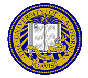# Outline for April 9, 1997

1. Greetings and Felicitations
1. For NTU: All homework is due 1 week after the due date; I'll put this explicitly on future assignments
2. Solving ax mod n = 1:
1. { r1, ...,rPHI(n)} reduced set of residues
2. by definition, { ar1 mod n, ..., arPHI(n) mod n} is same set
3. So, PI(ari mod n ) = PI ri (product from 1 to PHI(n))
4. This means (aPHI(n) mod n) PI ri = PI ri, giving aPHI(n) mod n = 1.
5. Ex: a = 5, n = 7; 5x mod 7 = 1; x = 5 PHI(7)-1 mod 7 = 55 mod 7 = 3.
3. Euclid's extended GCD algorithm
1. GCD(4, 6): remainder of 4 into 6 is 2; remainder of 2 into 4 is 0; GCD is 2
2. GCD(14, 22): remainder of 14 into 22 is 8; remainder of 8 into 14 is 6; remainder of 6 into 8 is 2; remainder of 2 into 6 is 0; GCD is 2
3. Extend: find x, y s.t. xa + yn = gcd(a, n) as then if GCD is 1, xa + yn = 1 and x solves ax mod n = 1.
4. Ex. 1: a = 5, n = 7
g0 = 7, x0 = 0, y0 = 1 (note g0 = 5x0 + 7y0);
g1 = 5, x1 = 1, y1 = 0 (note g1 = 5x1 + 7y1); q = g0/g1 = 1
g2 = 2 = 7 - 5q; x2 = 0 - 1q = -1; y2 = 1 - 0q = 1 (note g2 = 5x2 + 7y2); q = g1/g2 = 2;
g3 = 1 = 5 - 2q; x3 = 1 - (-1)2 = 3; y3 = 0 - (1)2 = -2 (note g3 = 5x3 + 7y3); q = g2/g3 = 0
GCD is x3, or 3.
5. Hence 5x mod 7 = 1 => x = 3, and 5(3) + 7(-2) = 15 - 14 = 1
4. ax mod n = b
1. Solve ax0 mod n = 1, then x = bx0 mod n
2. Ex: 5x mod 7 = 3; as x0 = 3, x = 3(3) mod 7 = 2
5. Chinese Remainder Theorem

1. Let g = gcd(a, n) and g divide b. Then ax mod n = b has g solutions of the form
x = [(b/g)x0 + t(n/g)] mod n,
where x0 solves (a/g)x mod (n/g) = 1.
2. Ex: solve 5x mod 21 = 1. 21 = 3(7); need x1, x2 such that
5x1 mod 3 = 1 and 5x2 mod 7 = 1; solns. x1 = 2, x2 = 3. So the solution to x mod 3 = 2 and x mod 7 = 3 also solves 5x mod 21 = 1
Technique: find y1: (21/3)y1 mod 3 = 1, so 7y1 mod 3 = 1, or y1 = 1
find y2: (21/7)y2 mod 7 = 1, so 3y2 mod 7 = 1, or y2 = 5
x = [(21/3)y1x1 + (21/7)y2x2] mod 21 = ((7)(1)(2) = (3)(5)(3)) mod 21 = 59 mod 21 = 17
6. Transposition ciphers
1. Show rail-fence cipher as example
2. Show anagramming

You can get this document in Postscript, ASCII text, or Framemaker version 5.1.
Notes by Christina Chang: [Postscript] [Text] [Microsoft Word]Send email to cs253@csif.cs.ucdavis.edu.

Department of Computer Science
University of California at Davis
Davis, CA 95616-8562